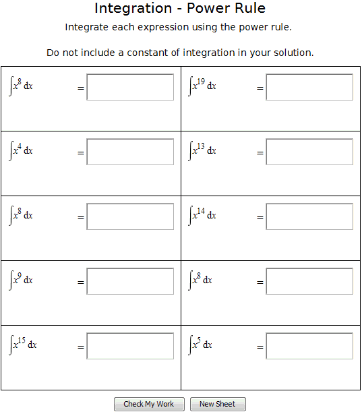New Features in Maple 2018 - Practice Sheets for Students - Maplesoft# Practice Sheets for Students

Student packages in Maple offer focused learning environments in which students can explore and reinforce fundamental concepts in the same way their instructor does in class. Student packages are available for a variety of core subjects, including calculus, precalculus, linear algebra, statistics, vector calculus, multivariate calculus, numerical analysis, and more. In Maple 2018, the Student Basics package had been expanded to give instructors a way to provide drill-and-practice exercises for students that Maple will grade automatically, giving the students immediate feedback.

### PracticeSheet

The new PracticeSheet command generates a grid of practice problems for drilling math. Practice sheets can test arithmetic, algebra, calculus, factorization and more, with each problem generated with randomized parameters.

Results can be instantly marked, and sheets updated with new problems.

 > with(Student:-Basics):

This practice sheet generates a new Maple worksheet that drills the student in adding randomly generated integers.

 > PracticeSheet("integer+integer");This practice sheet asks the student to integrate several expressions using the power rule.

 > PracticeSheet("%int(variable(x)^integer(range=2..20),variable(x))",  title="Integration - Power Rule",  message="Integrate each expression using the power rule. \n\nDo not include a constant of integration in your solution." );Prêt(e) pour l'étape suivante ?

*Ce programme d'évaluation n'est proposé actuellement ni aux étudiants ni aux particuliers.

Achetez: